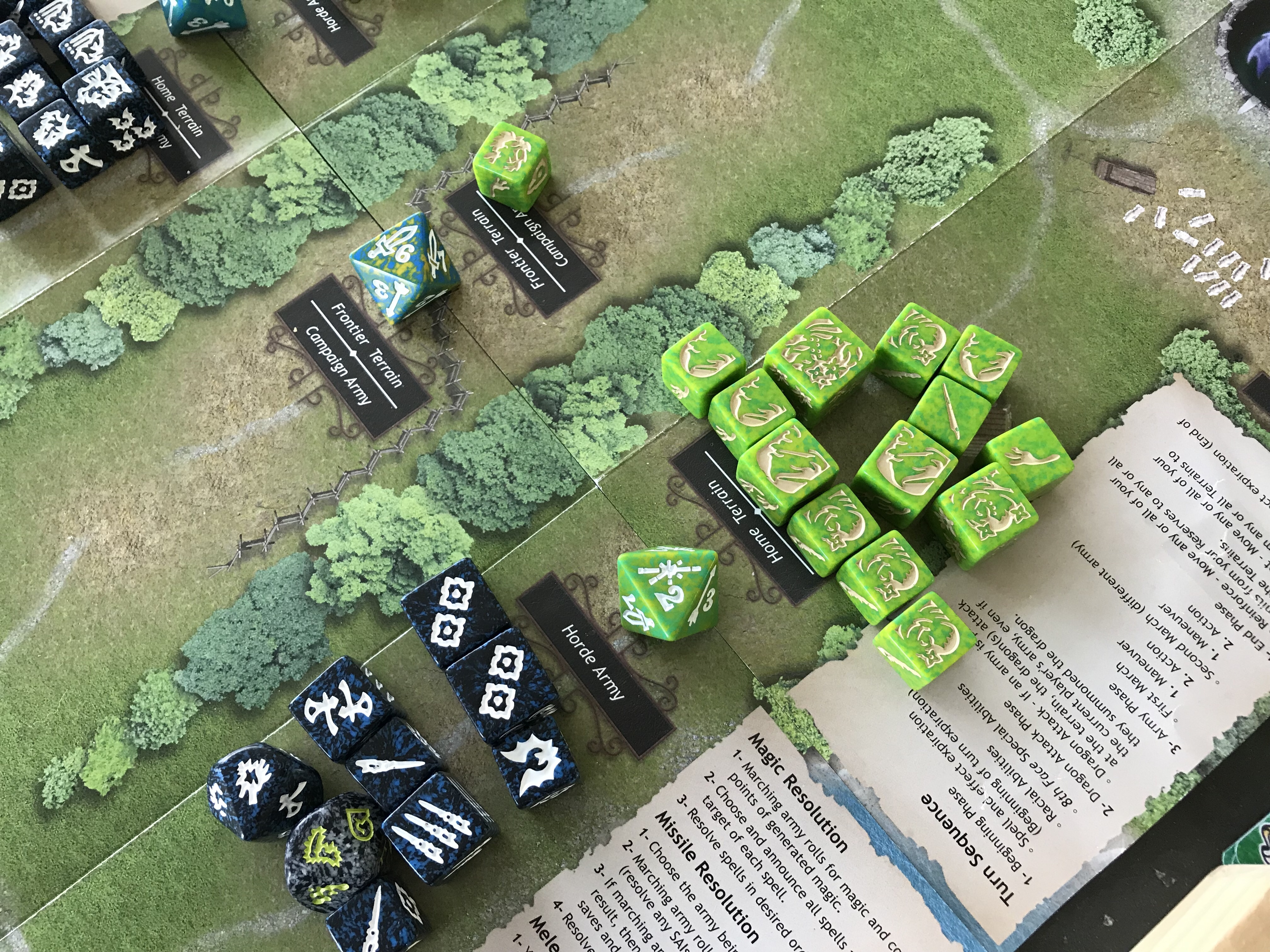Having recently bought a few Treefolk kickers, I thought that it would be a good idea to learn how they work before putting them into battle against my arch-rival and co-contributor to this blog, Nathan Miller.

As a lover of Spreadsheets, I set about producing one to carry out the calculations for the basic actions of each unit. I calculated the expected value for each action for each type of unit, and the corresponding Standard Deviation for each, both under normal circumstances and, in the case of Manoeuvres, I also looked at how the Rapid Growth racial ability assists in terrains with the Earth element.

Being the generous person that I am, I have decided to share the fruits of my travails with the readers of this blog, in the hope that they might find them useful.

#### Using the Tables

Using the tables to get useful results it easy. To get the expected value for an action for an army, simply add together the numbers in the ‘mean’ column.

e.g. I have a small 9 health army on a coastland terrain. Units are:-

1 x Pine

2 x Pineling

If I want to know the expected result for, say saves, for this army, I add together 1.0 + 0.833 + 0.833 + 0.333 + 0.333 = 3.333.

If I want the expected number of Manoeuvre results, I add 0.5 + 0.333 + 1.0 + 0.333 + 0.333 = 2.5

On a terrain containing the earth element (yellow), and we are counter-manoeuvring, the manoeuvres are read from the ‘Rapid Growth’ row, so our army would now get 0.917 + 0.611 + 1.5 + 0.556 + 0.556 = 4.14

As you are aware, the expected result is only one weapon that we have in our armoury. This tells us the ‘middle’ of all the values that we would get if we rolled these dice indefinitely, but it tells us nothing about the spread of our results. The standard deviation column tells us this.

For the non-statisticians amongst us, if there are any, one standard deviation either side of the mean gives us the boundaries for where 66% of our results will lie, and 95% of our results will fall between the mean minus two standard deviations and the mean plus two standard deviations.

e.g. I have an army of Darkwombles that have a melee expected value of 6 hits with a standard deviation of 1.5 – this tells me that I should be expecting 66% of my results to lie between 6-1.5 and 6+1.5 , in other words between 4.5 and 7.5 . Furthermore, I know that 95% of my results will be between 6 – 2 x 1.5 and 6 + 2 x 1.5 , i.e. 95% of the time I will score somewhere between 3 and 9 melee results for this particular army of Darkwombles.

Unfortunately, standard deviations do not simply add together like means (expected values) but, if you think of our old Greek friend Pythagoras, he gives us the answer.

To combine standard deviations, you simply square the numbers, add them together, and then take the square root. Exactly as we do to find the length of hypotenuse from the other sides of a right angled triangle.

The Standard Deviation for saves in the above army, therefore is:-

S.D. = SQRT ( 1.414 ^ 2 + 1.213 ^ 2 + 1.213 ^ 2 + 0.471 ^ 2 + 0.471 ^ 2 )

= SQRT ( 2 + 1.471 + 1.471 + 0.222+ 0.222 ) = SQRT( 5.386 ) = 2.32

This means that my 9 health army will produce between 3.333 – 3.32 and 3.333 + 3.32 ( between 0.013 and 6.653) two thirds of the time.

Taking this army on a terrain containing the earth element and looking at manoeuvres:-

S.D. = SQRT ( 1.382 ^ 2 + 0.921 ^ 2 + 0.866 ^ 2 + 0.497 ^ 2 + 0.497 ^ 2 )

= SQRT ( 1.910 + 0.848 + 0.75 + 0.247 + 0.247 ) = SQRT ( 4.002 ) = 2

So, this army will score between 2.14 and 6.14 manoeuvres, using Rapid Growth ability during counter-manoeuvre, 66% of the time.

If these tables are useful, please let me know and I can publish other races.

My Treefolk calculator spreadsheet, which does all the above calculations for you, can be downloaded from here:-